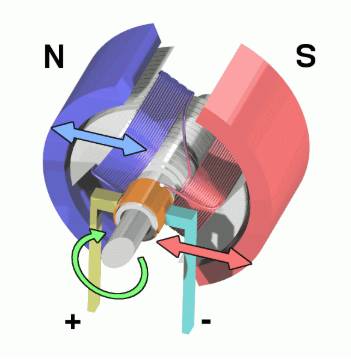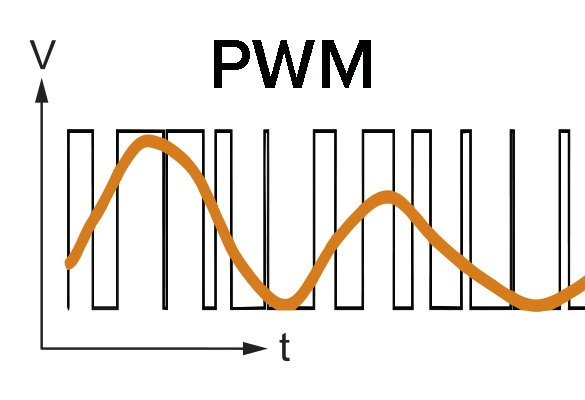## Analysis of the principle of PWM control motor speed

NEWS    |    Jan 15, 2023

The principle of DC motor is electric magnetism: the energized wire will generate a magnetic field (electromagnetic induction rotating magnetic field drives the rotor to rotate)

The motor is composed of a stator and a rotor. One generates a rotating magnetic field and the other is a magnetic pole. The rotor (bearing) of the motor rotates.

This realizes the conversion of electrical energy -> magnetic energy -> mechanical energy.

The following figure can be more intuitively understood:Principle of PWM

The speed of the motor is controlled by PWM, which is actually realized by controlling the magnitude of the power supply current.

The force that the current-carrying wire receives in the magnetic field is called Ampere's force, and the formula of Ampere's force: F=BIL.

Among them, F is the magnitude of the force, I is the magnitude of the current, and L is the length of the wire. Under the condition that other conditions remain unchanged, controlling the current passing through it means controlling the magnitude of the ampere force.The resistance R of the motor is basically constant, then the current I = U/R, F= BLU/R.

In the case that R B L remains unchanged, the essence of controlling the magnitude of the ampere force is to modify the magnitude of the supply voltage.

We also know that the essence of controlling the motor speed is to supply different power supply voltages to the motor. The higher the voltage, the faster the motor speed.

The essence of PWM is pulse width modulation, which converts DC voltage into analog signals of different voltage values by outputting different duty ratios.

Control motor speed

The duty cycle can realize the adjustment of the motor speed. We know that the duty cycle is the ratio of a cycle in the high-level state. The larger the ratio of the high-level, the larger the duty cycle.

For For DC motors, the motor can rotate when the output pin of the motor is at a high level. Since the inductance has the function of preventing the sudden change of the current, it will not stop, and will maintain the original speed. In this way, the speed of the motor is the average voltage value output in the cycle, so in essence, our speed regulation is to put the motor in a : In the state of full-speed rotation and non-full-speed rotation, then the average speed in one cycle is the speed adjusted by our duty cycle.

Summarize

In motor control, the greater the voltage, the faster the motor speed, and the output of different analog voltages through PWM can make the motor reach different output speeds.

Of course, in motor control, different motors have their own frequency. If the frequency is too low, the movement will be unstable. If the frequency is just within the hearing range of the human ear, sometimes whistling can be heard. The motor with too high frequency may not be able to respond, and the normal motor frequency is better between 6-16kHZ.

The output voltage is different, and the motor speed is different. Then we can know that the speed regulation of the motor can be realized by sliding the rheostat or changing the power supply of different voltages, but in practical applications, PWM is obviously more convenient.

The so-called PWM is a pulse width modulator, which provides the motor with an adjustable pulse width with a certain frequency through the modulator. The larger the pulse width is, the larger the duty cycle is, the larger the average voltage supplied to the motor is, and the higher the motor speed is. Conversely, the smaller the pulse width, the smaller the duty cycle. The smaller the average voltage supplied to the motor, the lower the motor speedBACK